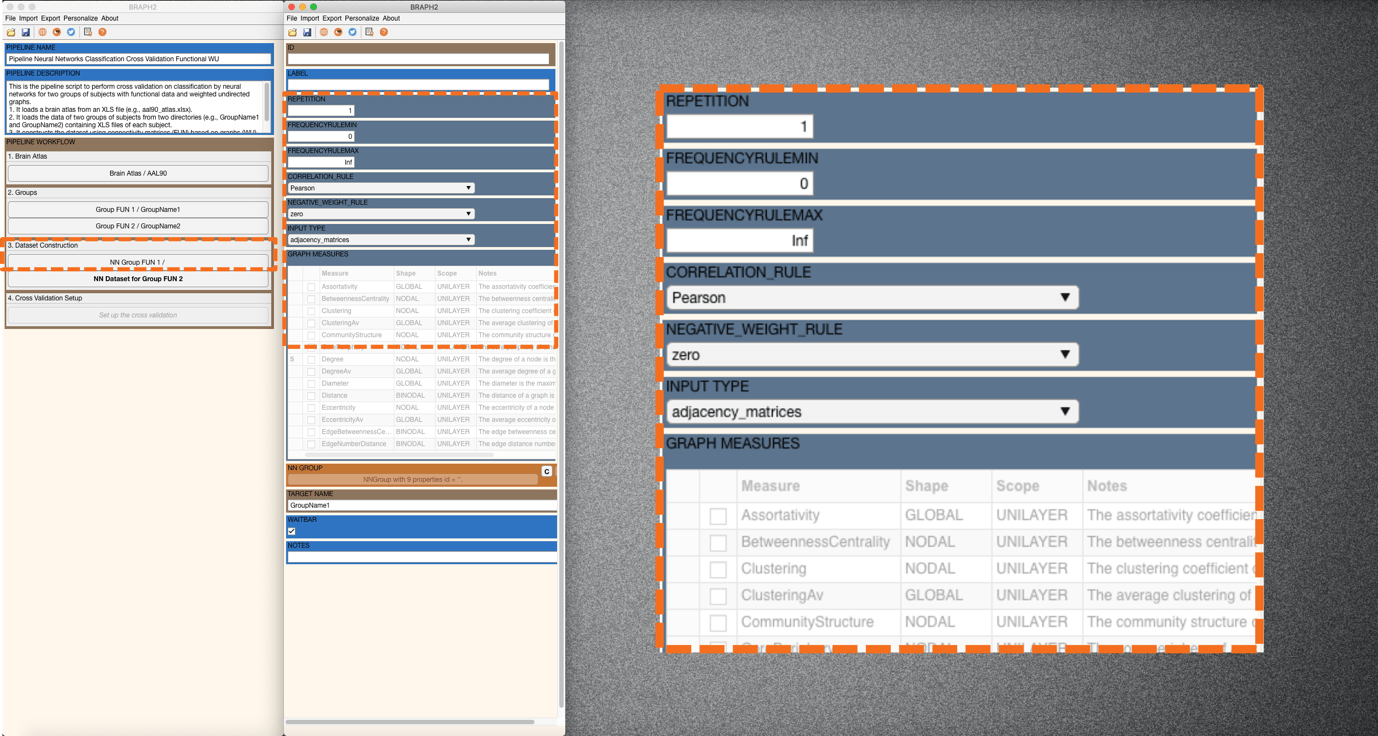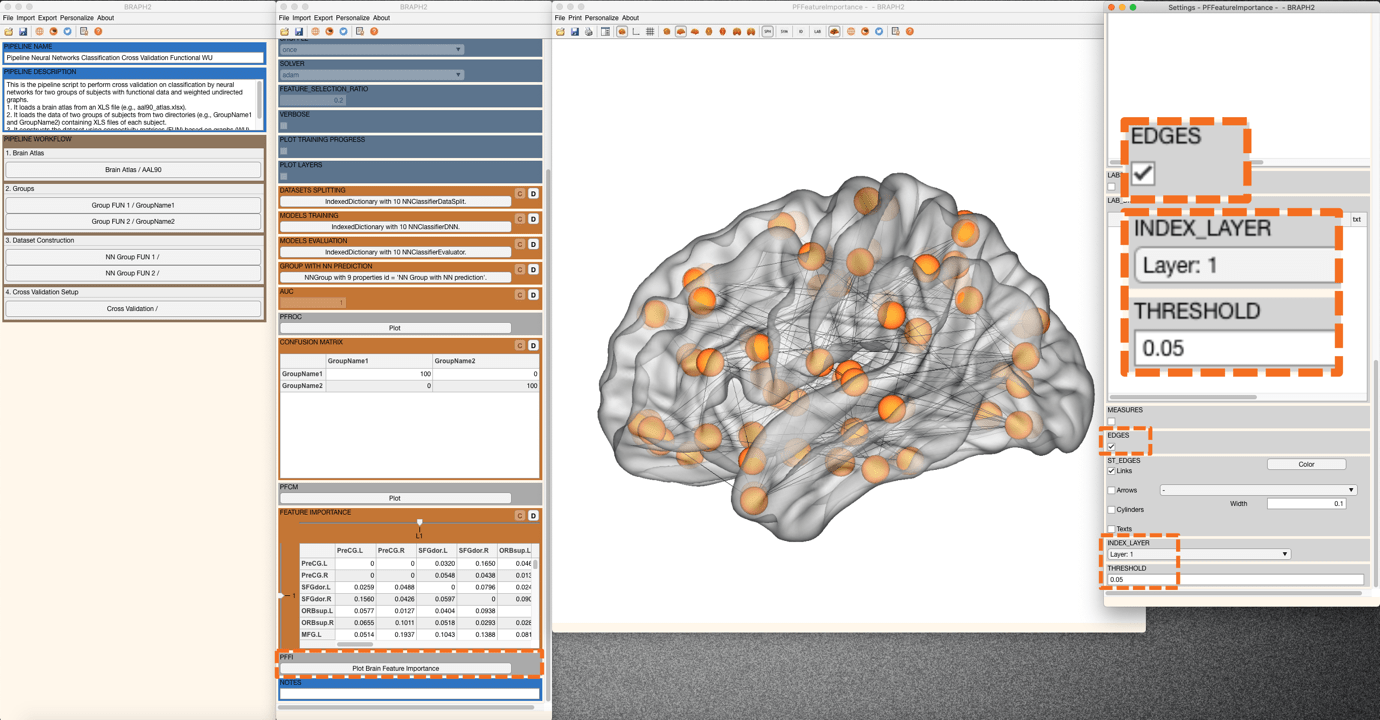# Pipeline Neural Networks Classification Cross Validation Functional WU

Description:

This is the Graphical User Interface (GUI) tutorial for the pipeline that performs cross-validation on a classification using neural networks of two groups of subjects with functional data and weighted undirected graphs.

In this tutorial, we will use the example data, but you can use your own data as well (for instructions on how to prepare your own functional data to be analyzed in BRAPH2, see Tutorial_data_format_braph2).

• Start BRAPH 2 and select the Pipeline from the main GUI

Start MATLAB, navigate to the BRAPH2 folder and run “braph2” with the following command:

>> braph2

Select the Pipeline Neural Networks Classification Cross Validation Functional WU in the right menu. You can use the search field, typing Neural Networks Classification for example.

The first step is to load a brain atlas from an XLS file. Press the button “Load a Brain Atlas XLS” (the only one active in the pipeline GUI) and then select the aal90_atlas.xlsx in the directory ./braph2/pipelines/functional NN /example data FUN (fMRI)/classification.

Finally, you can visualize the atlas by pressing “Plot the brain atlas” and playing with the surface settings. Check the Brain Atlas section from the tutorial of module 1 for more information on how to control the appearance of this interface.

To load the data for the two groups you would like to compare press “Load Group FUN 1 from XLS” and select the folder for subjects of Group1 which is at the directory ./braph2/pipelines/functional NN/example data FUN (fMRI)/classification/xls/GroupName1. After, load the data for Group 2 which can be found at the directory ./braph2/pipelines/functional NN/example data FUN (fMRI)/classification /xls/GroupName2.

• Dataset Construction

In this step we decide the input to use for the classification. In this case, we can choose between using adjacency matrices or graph measures.

To construct the dataset for the first group press “NN Dataset for Group 1” which will open a GUI to select the type of input data (adjacency matrices or graph measures). We select adjacency matrices as input (Figure 1), and then we press “C” to create the dataset.Figure 1. For INPUT TYPE we select adjacency matrices.

• Cross Validation Setup

In this step, we divide the data into a training set and a validation set, according to the number of K folds for the cross-validation. Then, these K models are trained and validated for the K folds iteratively with the selected parameters. Finally, we evaluate the average performance of the neural network models on all the validation sets.

Press “Set up the cross validation” in the main pipeline GUI. The first thing to do is to decide the parameters for training the K models. We change K FOLD from 5 to 10, and we also change the FEATURE SELECTION RATIO from 1 to 0.2 (20%) as can be seen in Figure 2. For the rest of the parameters, we leave the default options.Figure 2. Model parameters.

Descriptions for each option/parameter are illustrated below:

LAYERS: This parameter specifies (i) how many fully connected layers are there and (ii) how many neurons are there in each layer as a vector. For instance, “[100 50]” means that there are two layers: 100 neurons for the first layer, and 50 neurons for the second layer.

(Note. In this case, each fully connected layer is followed by a dropout layer with a given dropout rate of 0.5.)

BATCH: This parameter specifies the size of the batch for each training iteration (as a positive integer). A batch is a subset of the training set and is used to (i) evaluate the gradient of the loss function and (ii) update the weights.

EPOCHS: This parameter specifies the maximum number of epochs for training (as a positive integer). An epoch is the full pass of the training algorithm over the entire training set.

SHUFFLE: This parameter specifies the option for data shuffling. There are three options: “once”, “never”, and “every-epoch”. “once” means to shuffle the training and validation data once before training. “Never” means not to shuffle the data at all. “Every-epoch” means to shuffle the training data before each training epoch.

SOLVER: This parameter specifies the option of solver. There are three options: “sgdm”, “rmsprop”, and “adam”. “sgdm” uses the stochastic gradient descent with momentum (SGDM) optimizer. “rmsprop” uses the RMSProp optimizer. “adam” uses the Adam optimizer.

FEATURE SELECTION PROPORTION: This parameter specifies the proportion of the features to be selected for training the neural networks. All the features are analyzed and given individual scores based on the mutual information analysis. Based on the scores, all the features can be ranked, and the user can set a proportion for including part of the ranked features that are relatively informative.

VERBOSE: This parameter indicates whether to display training progress information in the command window.

PLOT TRAINING PROGRESS: This parameter indicates whether to create a figure and displays training metrics at every iteration.

PLOT LAYERS: This parameter indicates whether to create a figure to display the neural network architecture.

After setting the parameters, you can now train the K=10 models by clicking on the “C” in MODELS TRAINING (Figure 3).Figure 3. Model training and evaluation.

After training the model we evaluate its performance. Here we evaluate the performance on the average obtained from the validation sets. We can calculate GROUP WITH NN PREDICTION, AUC, and CONFUSION MATRIX and FEATURE IMPORTANCE (Figure 3).

In GROUP WITH NN PREDICTION, one can get a group in which subjects convey the prediction from the trained neural networks. In AUC, one can get the area under the receiver operating characteristic, a measure to evaluate the average performance from the K models when compared to actual target values. We can also plot of ROC curve by pressing PFROC.

In CONFUSION MATRIX, one can get the average confusion matrix determined by the target and predicted groups from all the validation sets. If you press the plot button in PFCM you can plot the confusion matrix.

Finally, in FEATURE IMPORTANCE, we can calculate the importance (from 0 to 1) of each connection for the prediction. We can also visualize it on a brain surface. For that, you can right-click on “Plot Brain Feature Importance”. A new figure with the brain surface will be prompted for brain region visualization (all with default settings). For further adjustments, go to settings and scroll down in the Settings panel. You will find EDGES panel and please tick on the box. By default, you will see the edges from the 0.05 (5%) most important connections (Figure 4).Figure 4. Feature importance plot, 5% most important connections.

We can change the threshold to see fewer edges, for example to 0.02, to see the 2% most important connections (Figure 5).Figure 5. Feature importance plot, 2% most important connections.